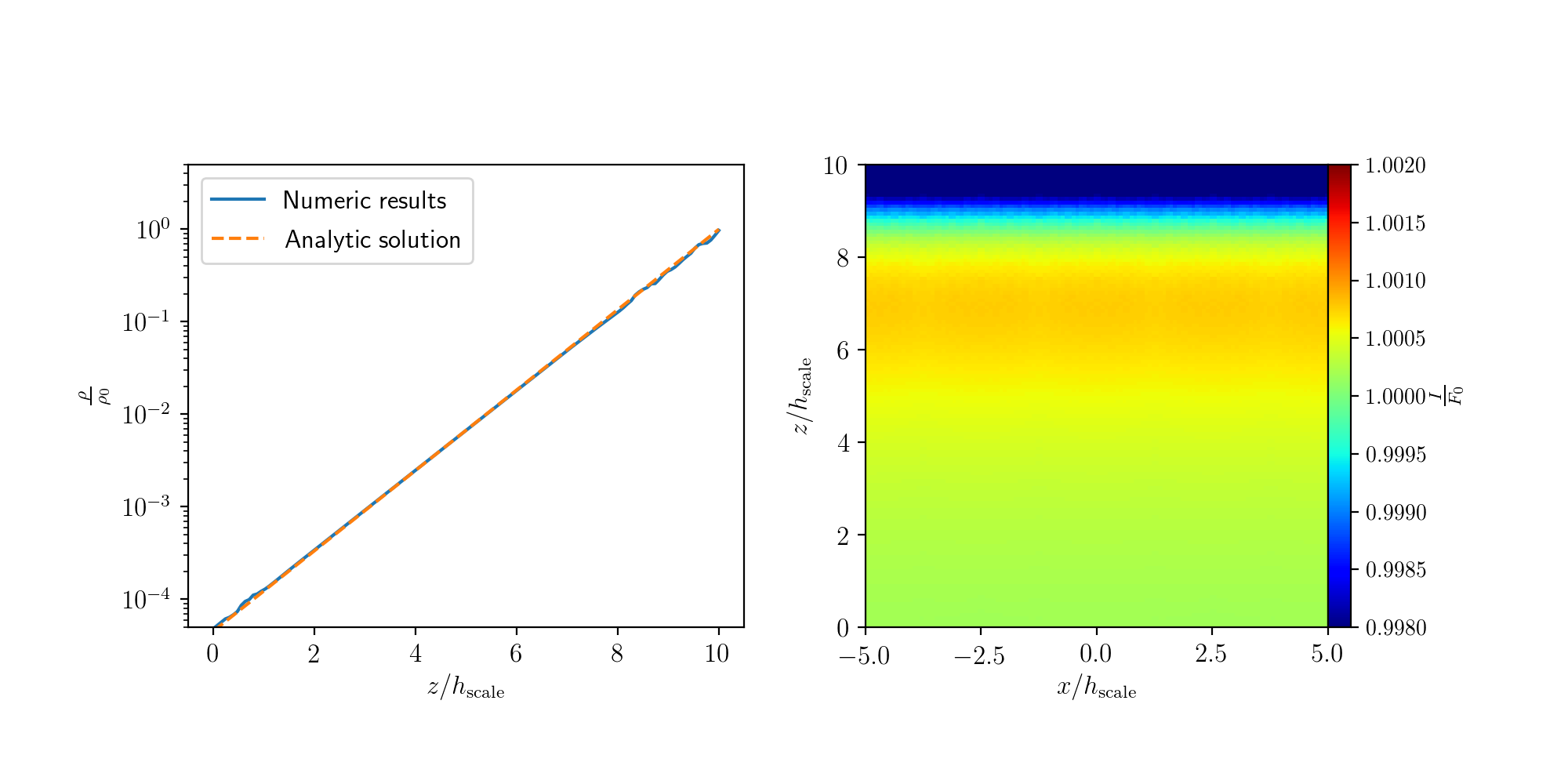#### Implementing Radiation Pressure into the AMR code FLASH

Radiation pressure has likely a huge impact on many astrophysical phenomena over a large range of scales. For example, on galactic scales, newly formed stars in ultra luminous infrared galaxies are suggested to set an upper boundary to the star formation rate through radiation. On parsec scales, radiation pressure is thought to be the primary feedback mechanism in massive star clusters, which governs the star formation efficiency of giant molecular clouds.
We extended the radiation transfer TreeRay module of FLASH by the ability to trace direct radiation pressure. Our extension includes extinction from dust and heating and cooling by radiation.
We performed various tests to study the new module extension. In one of these tests, we model the vertical structure of a gas subjected to plan-parallel radiation. The relative simplicity of this configuration enables us to find the analytic solution to the momentum equation in the limit of negligible extinction ($\tau \ll 1$), which reads $$\frac{\partial \rho \mathbf{v}}{\partial t} + \nabla \left( \left(P + P_\mathrm{rad} \right) \mathbb{1} \right) = \frac{\rho \kappa}{c}\mathbf{F},$$ and compare it to the results of the code.
The analytic solution in the hydrostatic equilibrium is an exponential profile, an analog to the density distribution in a homogeneous gravitational field. The vertical length-scale $h_\mathrm{scale}$ is determined by the initial incoming flux $F_0$. The gas behaves according to an adiabatic equation of state and, in addition, the gas is allowed to heat and cool via radiation. This keeps the gas close to the temperature $T_0 = \left(F_0/(ca)\right)^{1/4}$, where $c$ and $a$ are the speed of light in vacuum and the radiation constant, respectively. As the gas approaches its true thermal equilibrium, additional pressure gradients result in the growth of instabilities. The figure demonstrates that the new Flash module reproduces faithfully the analytic hydrostatic equilibrium.Figure: Vertical density profile (left panel) and local heating intensity (right panel) for a model of plan-parallel radiation and optically thin ($\tau \ll 1$) medium. The radiation is coming from the negative $z$-direction. The model has periodic boundary conditions in the $x$- and $y$-direction, and outflow and reflecting conditions in the positive and negative $z$ directions, respectively. The density evolves close to the analytic exponential profile (the analytic solution is indicated by a dashed line). The right panel shows that the local heating intensity is plan-parallel to the edge, thus it follows well the periodic boundary conditions.